Wiener-Khintchine Theorem

Recall the definition of the Autocorrelation functionof a function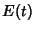,(1)

Also recall that the Fourier Transform ofis defined by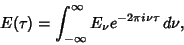(2)

giving a Complex Conjugate of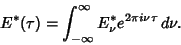(3)

Pluggingand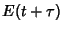into the Autocorrelation function therefore gives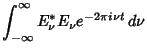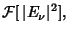(4)

so, amazingly, the Autocorrelation is simply given by the Fourier Transform of the Absolute Square of,(5)

The Wiener-Khintchine theorem is a special case of the Cross-Correlation Theorem with.